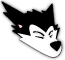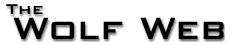User not logged in - login - register
 Home Calendar Books School Tool Photo Gallery Message Boards Users Statistics Advertise Site Info
go to bottom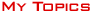|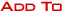|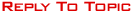Message Boards » » MA 132 lesson 7 help Page 
 mgarga32New Recruit1 Postsuser infoedit post I'm not sure how to get the numbers for the two empty columns on this problem. If anyone could tell me how or what formula to use that would be great!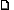10/17/2014 6:16:25 PM lewisjeAll American9196 Postsuser infoedit post This is the method most commonly known simply as "Euler's Method" (yes you could just Google it but I'm being nicer than that): http://mathworld.wolfram.com/EulerForwardMethod.htmlIf the differential equation is of the form y'=f(x,y), h is the step-size, (x0,y0) is the initial condition, and (x_n,y_n) is the nth approximation, then x_(n+1)=x_n+h, and y_(n+1)=y_n+h*f(x_n,y_n).Below I will show you how to calculate the approximations using Euler's Method, using h=dt=5 (this problem uses t instead of x, and dt instead of h); when getting the later solutions, you will get the earlier solutions.When t=5, n=t/h=1, and when t=20, n=t/h=4; this means we will need y1 and y4 for the first two solutions (you can figure out the rest).f(t,y)=-1/14*(y-70), t0=0, and y0=201.Then y1=y0+h*f(t0,y0)=201+5*(-1/14*(201-70))=2159/14, and t1=t0+h=0+5=5.You can keep going like this, but it's probably easier to use a spreadsheet.You can enter this into Excel or LibreOffice with a formula like this (it's easy in this case because f(t,y) does not actually depend on t), to more easily get the solutions; after entering 201 into A1, 0 into B1, and 5 into C1, place the formula =B1+C\$1 into B2, place =A1+C\$1*(-1/14)*(A1-70) into A2, and then select A2 and B2 and drag down to get the other solutions (look for the rows where column B has values 5, 20, 30, and 60). When you've read them off, you can change C1 to 1 and it will re-calculate the approximations using Euler's Method.10/18/2014 5:58:37 AM
Message Boards » Study Hall » MA 132 lesson 7 help Page 
go to top||Admin Options : move topic | lock topic